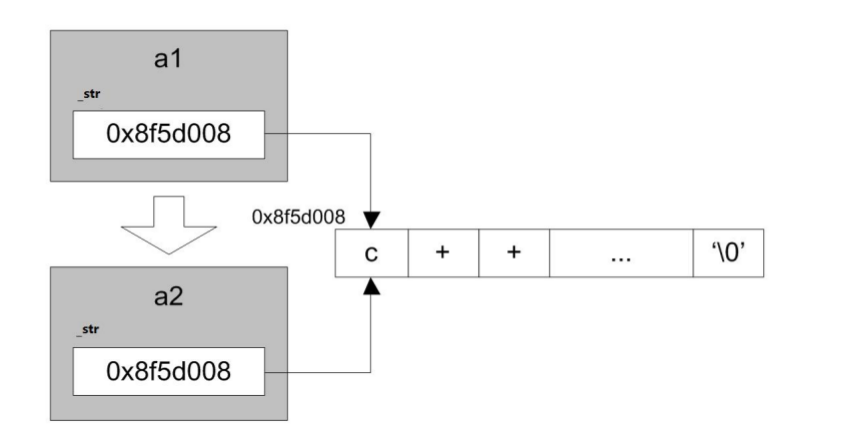# C++之封装

C++的三大特性就是封装、继承、多态。今天说说第一个封装。

``````class A  //定义了一个类，类名是A
{

}；``````

public公有可访问可访问
private私有可访问不可访问

``````bool Stack::isFull()   //成员函数  在类外写要写上类名：：
{
}``````

## 1、构造器

``````class 类名
{
类名(形式参数)
构造体
};
//实例就像下面这样，A为类名
class A
{
A(形参)
{}
};``````

1 与类名同， 在对象创建时自动调用,完成初始化相关工作。

2 无返回值， 可带参数， 可以重载， 可默认参数。

3 默认无参空实现体， 一经自实现， 默认不复存在

``````#include <iostream>
#include <string.h>
using namespace std;

class Stack
{
public:
Stack()                         //构造器
{
top = 0;
space = new char;
memset(space,0,1024);
spaceSize = 1024;
}

Stack(int size)                    //构造器重载，这里这种情况不能写为默认参数，
//不然用stack s创建对象的时候，会报错，二义性
//如果没有上边的那个构造器，就应该写上默认参数
{
top = 0;
space = new char[size];
memset(space,0,size);
spaceSize = size;
}

//initial list  初始化列表 完成初始化(数据成员的)  效率很高
//初始化列表实始化顺序, 跟列表中的顺序无关.实始化顺序和private中写的顺序一样
//此列表不要拿被初始化的成员去初始化其它
Stack(int size = 1024)
:top(0),
spaceSize(size),
space(new char[size]{0})
{
}

bool isFull();

private:
int  top;
int  spaceSize;
char *space;
};

bool Stack::isFull()   //成员函数  在类外写要写上类名：：
{
}
int main()
{
Stack s;          //创建对象
cout<<s.isFll()<<endl;//成员函数的调用，用.

Stack s2(10);
cout<<s2.isFull()<<endl;
return 0;
}
``````

## 2、析构器

``````class 类名
{
~类名()
析构体
}``````

1 、对象销毁时， 自动调用， 完成销毁的善后工作。

2 、无返值， 与类名同， 无参， 不可以重载与默认参数。

3 、系统提供默认空析构器， 一经自实现， 不复存在。

``````class Stack
{
public:
Stack()                         //构造器
{
top = 0;
space = new char;
memset(space,0,1024);
spaceSize = 1024;
}

~Stack()
{
delete []space;
}
};``````

## 3、拷贝构造器

``````class 类名
{
类名(const 类名 & another)
{
拷贝构造体
}

}；``````

1、系统提供默认的拷贝构造器。一经实现,不复存在。

2、系统提供的是等位拷贝,也就是所谓的"浅浅"的拷贝。

3、要实现深拷贝,必须要自定义。

4、发生时机:制作对象的副本,以对象作为参数和返回值。

### 浅(shallow)/深(deep)拷贝

``````class Date
{
public:
Date(int y = 2016,int m =6 ,int d = 6)
:year(y),month(m),day(d){}    //构造器，初始化列表

Date(const Date &another)   //拷贝构造器，浅拷贝
{
year = another.year;
month = another.month;
day= another.day;
}
void dis()
{
cout<<"year:"<<year
<<"month"<<month
<<"day"<<day<<endl;
}
private:
int year;
int month;
int day;
};

int main()
{
Date d(2016,8,8);  //调用构造器
d.dis();

Date dd(d);            //调用的是拷贝构造器
return 0;
}````````````#include <iostream>
#include <string.h>
using namespace std;
class DataStr
{
public:
DataStr()                          //构造器
{
cout<<"constructor"<<endl;
_str = new char;
strcpy(_str,"C++ is the best language in the World");
}

DataStr(const DataStr & another)  //深拷贝构造器
{
cout<<"copy constructor"<<endl;
_str = new char[strlen(another._str)+1]; //创建新的堆空间，分配内存 注意strlen求的长度
strcpy(_str,another._str);               //将传入的another对象内容拷贝一份给新的
}

~DataStr()                    //析构器
{
cout<<"destructor"<<endl;
delete []_str;
}

void dis()
{
cout<<_str<<endl;
}

private:
char *_str;
};
int main()
{
DataStr ds;
ds.dis();

DataStr ds2 = ds; //调用深拷贝构造器
return 0;
}``````

## 4、this 指针

this指针就是指向调用对象的指针。系统在创建对象时,默认生成的指向当前对象的指针。这样作的目的,就是为了带来使用上的方便。

this 指针的使用规则

1、指向当前对象,可用于用所有的成员函数,但不能应用于初始化列表。

2、this 是以隐含参数的形式传入,而非成员的一部分,所以不会影响 sizeof(obj)对象的大小。

3、this 指针本身是不能更改指向的,即,是 const 类型修饰的。

this 作用

1、指向当前对象,避免入参与成员名相同

2、支持基于返回 this 引用的多重串联调用的函数(连续赋值)。

``````#include <iostream>
using namespace std;
class Stu
{
public:
Stu(string name, int age)
{
this->name = name;//name = name;错误写法
this->age = age;
}

Stu & growUp()            //注意这里返回引用
{
this->age++;
return *this;    //这行代码可以实现串联调用，例如下边的
//s.growUp().growUp().growUp().growUp().growUp();这样使用
}

void display()
{
cout<<name<<" : "<<age<<endl;
}

private:
string name;
int age;
};

int main()
{
Stu s("www.mengchao.xyz",18);
s.growUp().growUp().growUp().growUp().growUp();
s.display();
return 0;
}``````

## 5、运算符重载

``````struct Comp
{
float real;
float image;
};
Comp operator+(Comp one, Comp another) //重载了+运算符
{
one.real += another.real;
one.image += another.image;
return one;
}
int main()
{
Comp c = {1.0,2.0};
Comp c2 = {3,4};
Comp sum = c1+c2; //operator+(c1,c2)可以直接使用重载的+号
return 0;
}``````

### 类中重载赋值运算符

``````class 类名
{
类名& operator=(const 类名& 源对象)
{
拷贝体
}

};``````
``````class A
{
A& operator=(const A& another)
{
//函数体
return *this;
}
};
``````

operator=的特性

1、系统提供默认的赋值运算符重载,一经自实现,不复存在。

2、系统提供的也是等位拷贝,也就浅拷贝,会造成内存泄漏,重析构。要实现"深深"的赋值,必须自定义。

3、自实现需要解决的问题有 3 个:1 重析构 (老问题) 2 内存泄漏 3 自赋值

4、返回引用,通常不能用 const 修饰。string a,b,c; (a= b) = c; (a+b) = c

### 浅赋值运算符重载

``````class Date
{
public:
Date(int y = 2016,int m =6 ,int d = 6)
:year(y),month(m),day(d){}

Date& operator=(const Date& another) //浅重载赋值运算符
{
this->year = another.year;
this->month = another.month;
this->day = another.day;
return *this;
}
private:
int year;
int month;
int day;
};``````

### 深赋值运算符重载

``````mystring & operator =(const mystring & another)
{
if(this == &another)
return *this;            //实现自赋值

delete []this->_str;        //解决内存泄露，先销毁掉原来的空间

int len = strlen(another._str);//解决重析构double free问题
_str = new char [len+1];
strcpy(_str,another._str);

return *this;   //实现连续赋值
}``````

## 6、栈对象返回

C++中的栈对象可以返回，但是不可以返回栈对象的引用。

返回对象会涉及到生成返回对象的副本，这事调用函数的程序可以使用的副本，因此，返回对象的时间成本包括了调用复制构造函数来生成副本所需的时间和调用析构函数删除副本所需的时间。返回引用可以节省时间和内存。直接返回对象与按值传递对象类似，他们都生成临时副本。同样，返回引用与按引用传递对象类似，调用和被调用的函数对同一个对象进行操作。

## 7、对象数组

``````#include <iostream>
using namespace std;
class Stu
{
public:
Stu(string n = ""):_name(n){}
void dis()
{
cout<<_name<<endl;
}

private:
string _name;
};
int main()
{
Stu s1= {Stu("zhangsan"),Stu("lisi")};
Stu s2[]= {Stu("zhangsan"),Stu("lisi")};
Stu * ps = new Stu{Stu("zhangsan")};
return 0;
}``````
Last modification：October 6th, 2019 at 02:11 pm